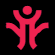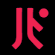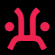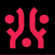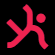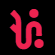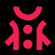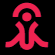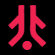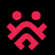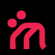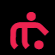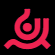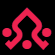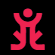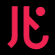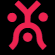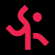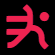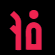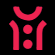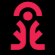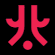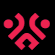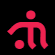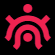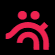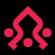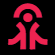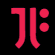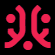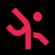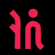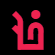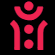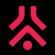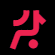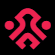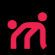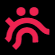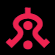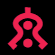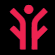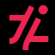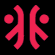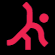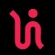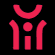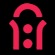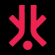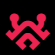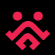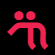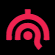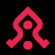## On the Subject of The Bioscanner

Initiating Security Protocol Angry Salamanders Sifting.

• Among your crew is an alien disguised as a crewmember. Find out who they are by completing the bioscan.
• Find three glyphs in the table below by doing the following:
• Turn the first letter of the serial number into a number, with A = 1. Modulo this by five, treating 0s as 5s.
• Pair this with the first digit of the serial number multiplied by the sum of batteries and ports modulo 11 and add 1.
• Turn the last letter of the serial number into a number, with A = 1, and modulo by 5, treating 0s as 5s.
• Pair this with the last digit of the serial number multiplied by the amount of indicators, modulo 11 and then adding 1.
• The third coordinate can be found by getting the first port present on the bomb reverse alphabetically and turning its occurrence rate (plus one) into a letter and pair this with the amount of batteries modulo 11 and add 1.
• If there are no ports, the column is A.
• A letter represents a column from left to right (A-E) while the number represents the row from top to bottom (1-11).
• If any coordinate is duplicated, add one to the number component of the second duplicated coordinate.
• The term modulo means to rapidly subtract by the number given, until the result is less than the number you are subtracting by.
• Press anywhere on the module to begin a seventy second sequence in where you must push the glyphs you discovered.
• Shift each glyph to the right by the number shown when the Bioscanner is activated wrapping to the next row if necessary.